# How Many Bundles of Shingles Do I Need?

When tackling any shingle roofing project, it's important to know ahead of time the amount of materials you will need. There's nothing worse than getting to the day of a build and realizing you are short material and scrambling to find the necessary items to get the job done. Calculating the needed material ahead of time and factoring in the projected waste will save you hours or even days of headaches.

## What is a Square?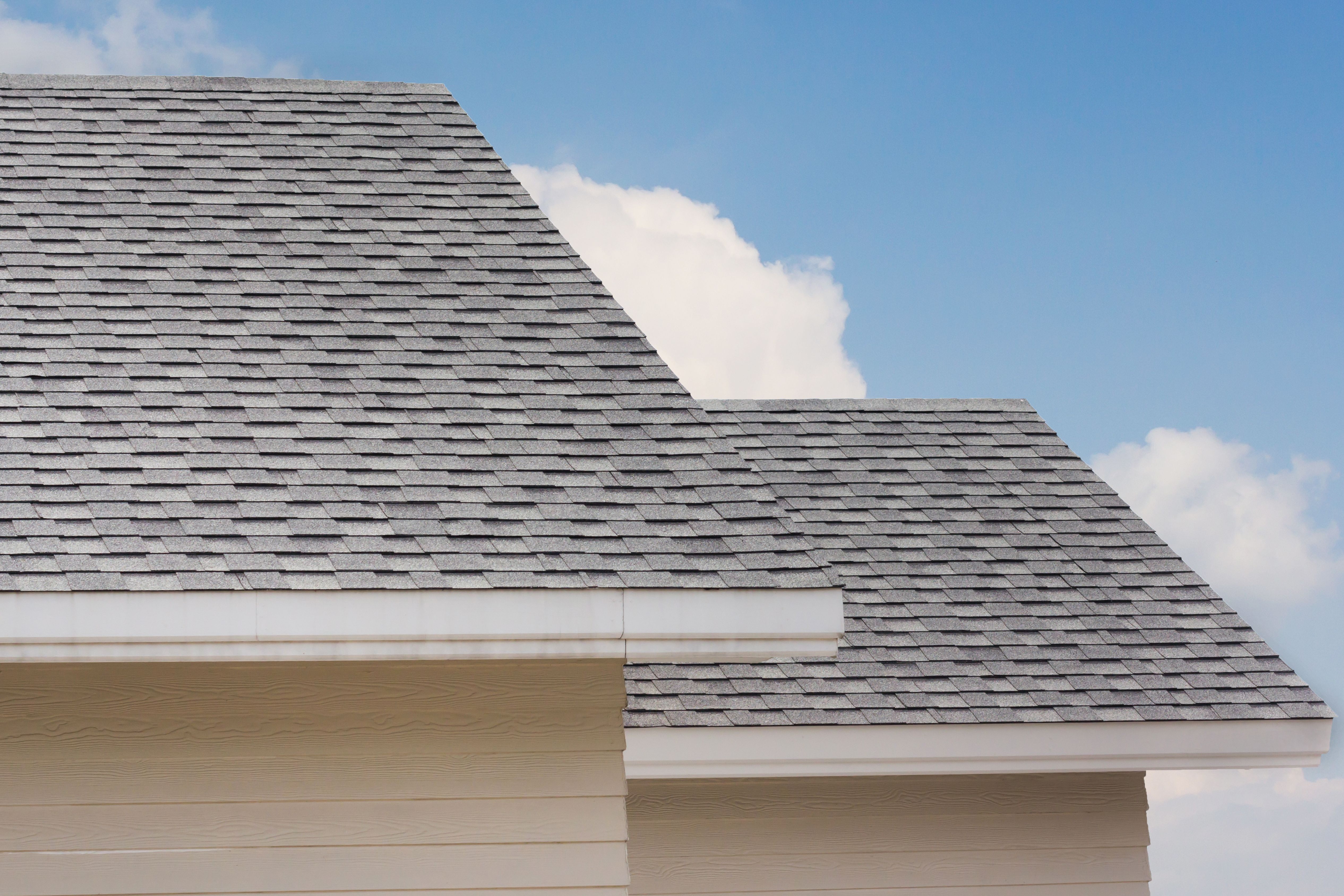The first step is to figure out the square footage of your roof's surfaces which will involve a little math. Depending on the complexity of your roof this can range from a simple task to a complex combination of equations. For the purposes of this article let’s just keep it simple and look at a two plane gable roof system.

We were all taught this in high school, but the area of a surface is figured by multiplying the length by the width. If you do this for both sides of the roof and add them together you will find your total square footage of the roof.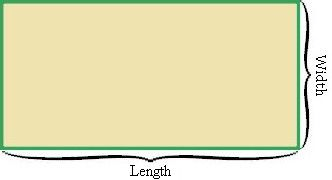With this information in hand, we can then start to calculate how many “squares” of shingles we will need. In roofer-talk, 1 square translate to 100 square feet. So for an easy example lets say we have 2 seperate roof slopes that are 1000 sqaure feet each. That would give us 20 squares. At Prostruct we created a simple calculator to estimate the amount of squares a given roof is by using the liveable square footage.

## The Rule of Three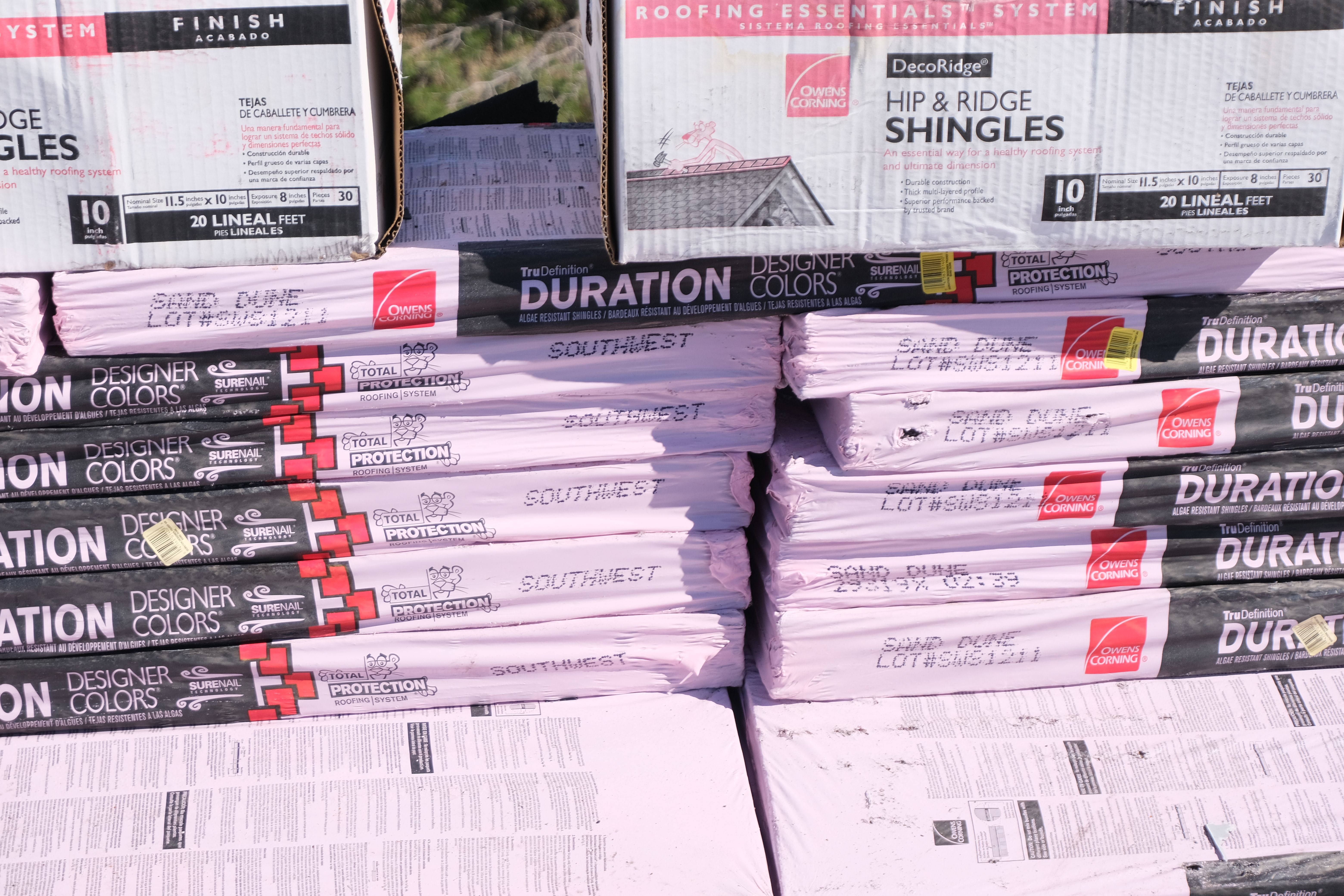Now enters the rule of three. For every one square, three bundles of shingles are required to cover the area. This is true for around 90% of shingle types and manufacturers, but you will want to double check when ordering that this is the case. Keep in mind this does not account for waste yet, which we will get into in a second. So if we go back to our example of a 2000 square foot roof, that would give us a total of 20 squares and require 60 bundles of shingles.

## Waste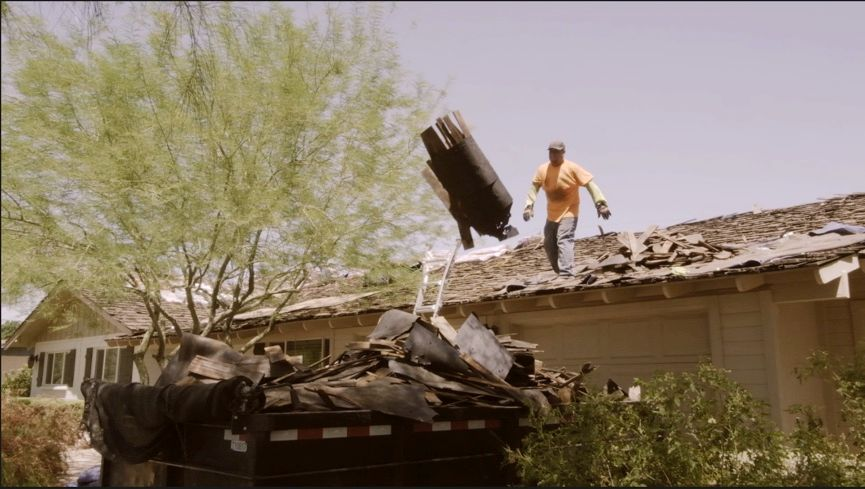This equation works perfectly if you were to use every inch of product and have no waste left over, unfortunately that is never the case. A good rule of thumb for estimating waste is 10%. So with the waste included in this, we have our 20 square roof, requring 60 bundles with 10% waste equaling 66 total bundles needed for the job.

## Underlayment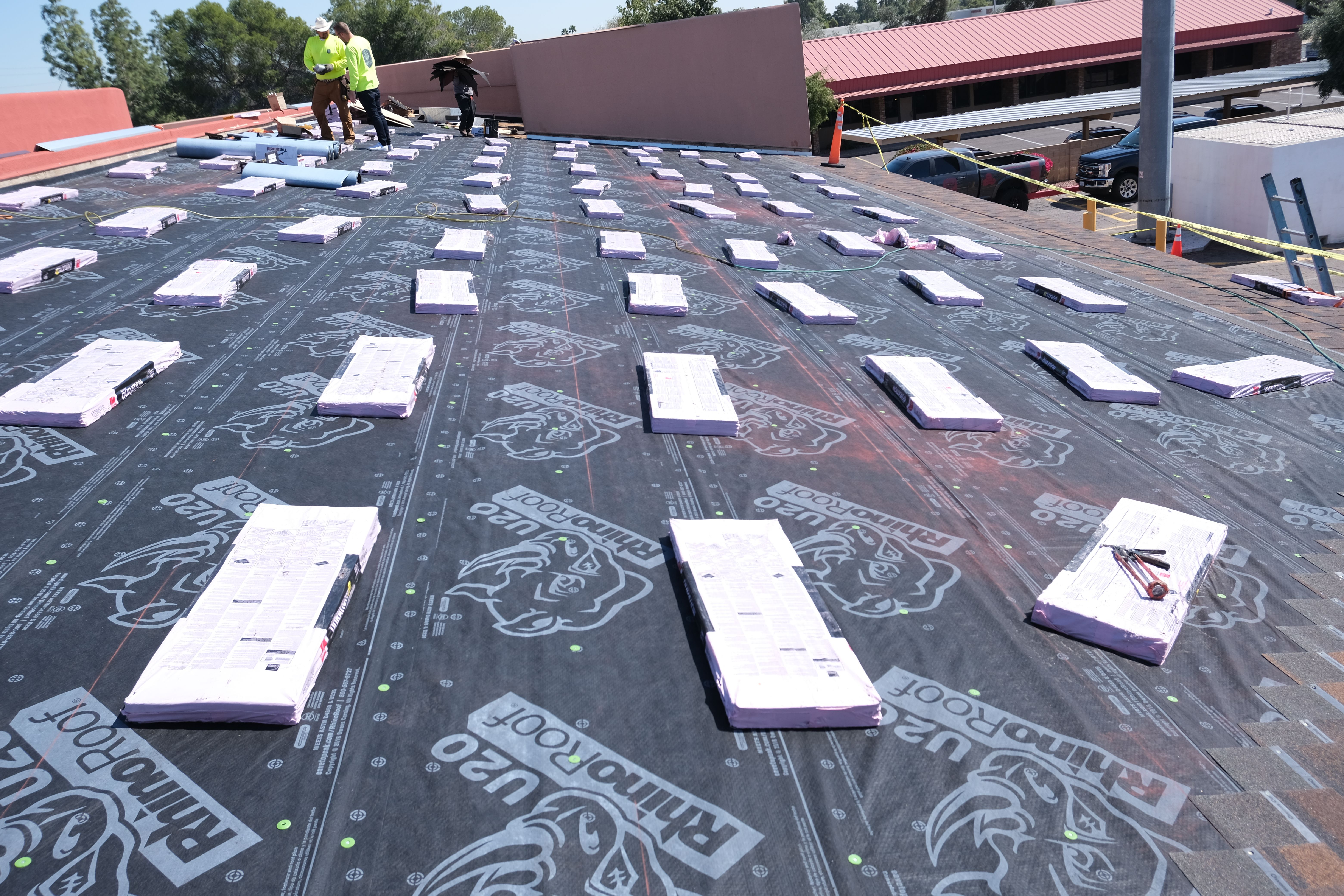When installing a new roof (not applying shingles directly on top of existing asphalt roof) you will also need the same amount of underlayment to create a barrier between the wood and the shingles. Underlayment usually comes in rolls covering 4 squares per roll. Returning to our example of a 2000 square foot roof, this would require 5 rolls of underlayment for the 20 square job.